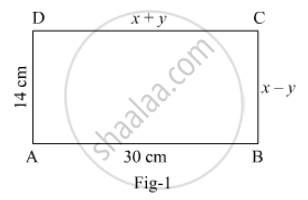# In Fig. 1, Abcd is a Rectangle. Find the Value Of X And Y. - Mathematics

In Fig. 1, ABCD is a rectangle. Find the value of x and y.#### Solution

In rectangle ABCD, DC = AB and BC = AD

⇒ x + y = 30 .....(i) and x − y = 14 .....(ii)

Adding (i) and (ii), we get

2x = 44

​⇒ x = 22

Put x = 22 in (i), we get y = 8

Hence, x = 22 and y = 8.

Concept: Equations Reducible to a Pair of Linear Equations in Two Variables
Is there an error in this question or solution?